# Test: Second Order Derivatives

## 10 Questions MCQ Test Mathematics (Maths) Class 12 | Test: Second Order Derivatives

Description
This mock test of Test: Second Order Derivatives for JEE helps you for every JEE entrance exam. This contains 10 Multiple Choice Questions for JEE Test: Second Order Derivatives (mcq) to study with solutions a complete question bank. The solved questions answers in this Test: Second Order Derivatives quiz give you a good mix of easy questions and tough questions. JEE students definitely take this Test: Second Order Derivatives exercise for a better result in the exam. You can find other Test: Second Order Derivatives extra questions, long questions & short questions for JEE on EduRev as well by searching above.
QUESTION: 1

### If y = tan-1 x, then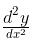in terms of y

Solution:

y = tan-1 x
=> tan y = tan(tan-1 x)
x = tan y
sec2 y dy/dx = 1
=> 1/(cos2y) dy/dx = 1
dy/dx = cos2y
Differentiate it with respect to x
d2y/dx2 = 2cos y(-sin y)(dy/dx)..................(1)
Put the value of dy/dx in eq(1)
=> d2y/dx2 = 2cos y(-sin y)(cos2 y)
=> d2y/dx2 = 2cos3 y siny

QUESTION: 2

###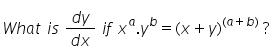Solution:

xa yb = (x−y)a+b
taking log both the sides.
log(xa yb)=log(x−y)(a+b)
alogx+blogy=(a+b)log(x−y)
differentiating both sides w.r.t 'x'.
(a/x+b/y)dy/dx = (a+b)/(x−y)[1−dy/dx]
dy/dx[b/y+(a+b)/(x−y)] = (a+b)/(x−y) − a/x
dy/dx[(bx−by+ay+by)/y(x−y)] = (ax+bx−ax+ay)/x(x−y)
dy/dx[(bx+ay)/y]=(bx+ay)/x
dy/dx = y/x

QUESTION: 3

### If f(x) = x + cot x,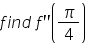​

Solution:

f(x) = x + cot x
f’(x) = 1 + (-cosec2 x)
f”(x) = 0 - 2cosec x(-cosec x cot x)
= 2 cosec2 x cot x
f”(π/4) = 2 cosec2 (π/4) cot(π/4)
= 2 [(2)^½]2 (1)
= 4

QUESTION: 4

Find the differential coefficient y = (sec 5x)5x

Solution:
QUESTION: 5

Differentiate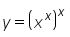Solution:
QUESTION: 6

If ey(x+5) = 1, then evaluateSolution:

ey =1/ (x+5)
Taking log both side we get
log ey = log {1/(5+x)}
then, y = log {1/(5+x)}
Differentiating both side ,
dy/dx =( x+5) . {-1/(5+x)²}
dy/dx = -1/(5+x) ……..( 1)
Again Differentiating, d²y/dx²= 1/(5+x)²
d²y/dx²= {-1/(5+x)}²
From equation (1)
d²y/dx² = {dy/dx}²

QUESTION: 7

Findfrom x = 2at2, y = at4

Solution:

y = at4,     x = 2at2
dy/dt = 4at3        dx/dt = 4at    => dt/dx = 1/4at
Divide dy/dt by dx/dt, we get
dy/dx = t2
d2 y/dx2 = 2t dt/dx……………….(1)
Put the value of dt/dx in eq(1)
d2 y /dx2 = 2t(1/4at)
= 1/2a

QUESTION: 8

The differential coefficient of tan-1 (log x) with respect to x is :

Solution:
QUESTION: 9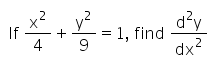Solution:
QUESTION: 10

Find the second derivative of excosx​

Solution: